# Analyzing On-Chip Interconnect with Modern C++

Oct. 16, 2014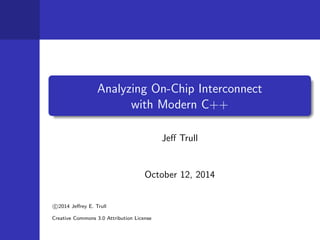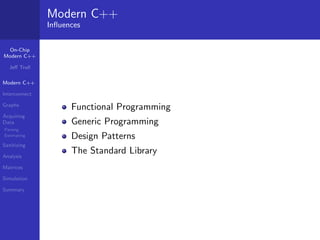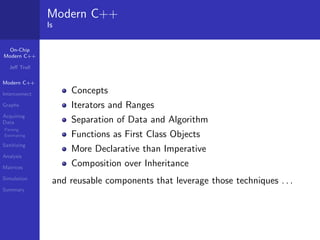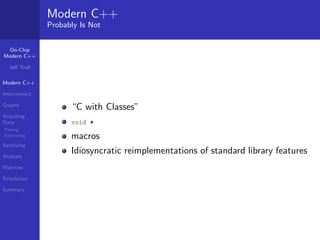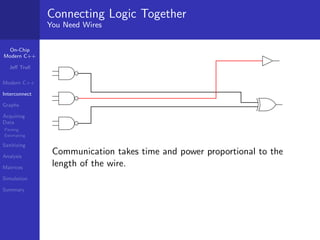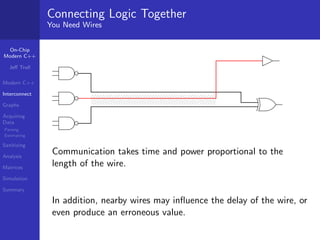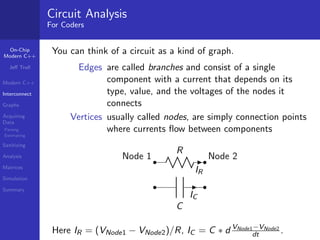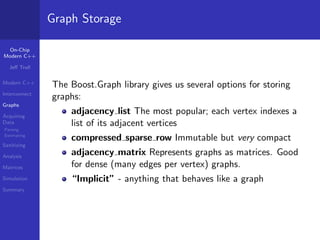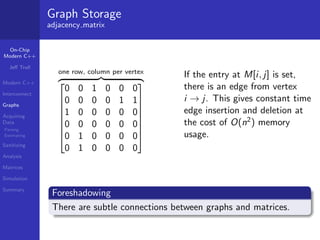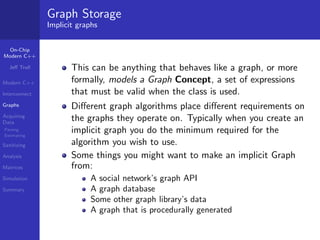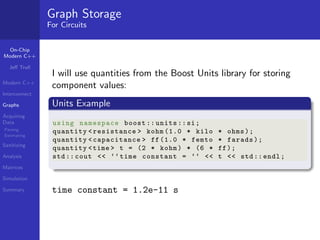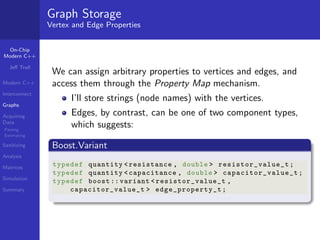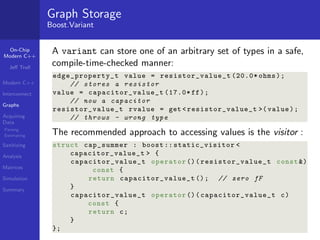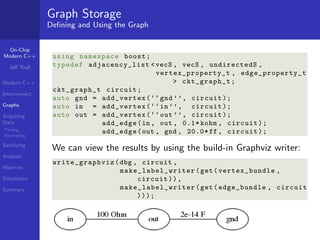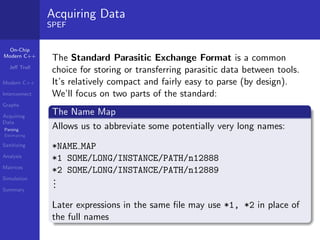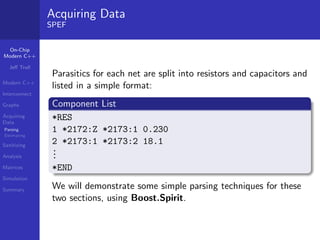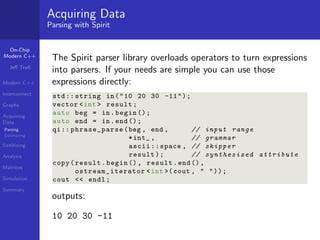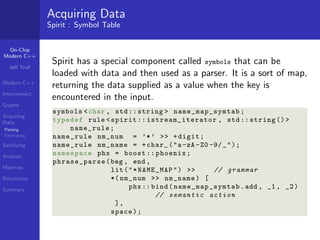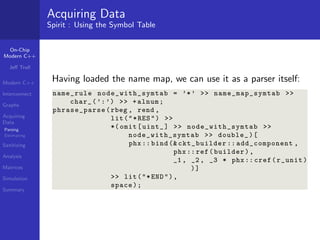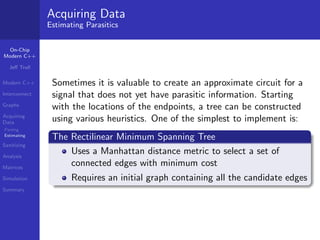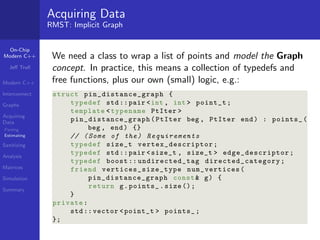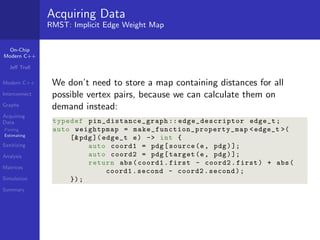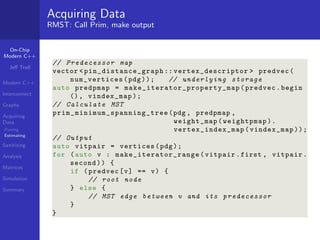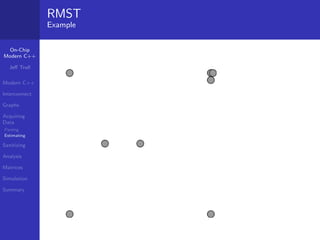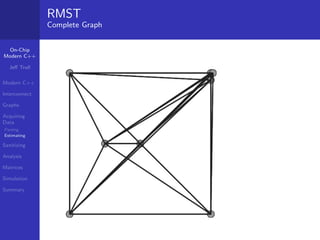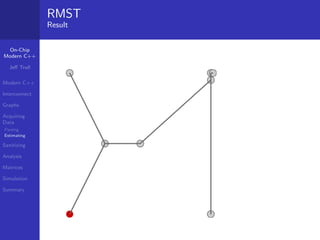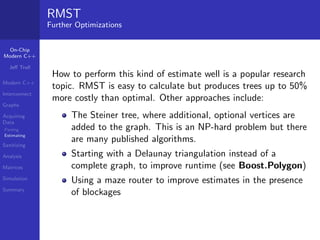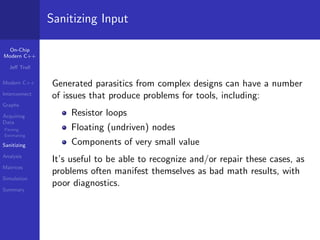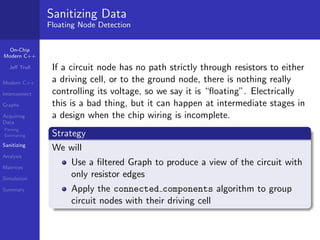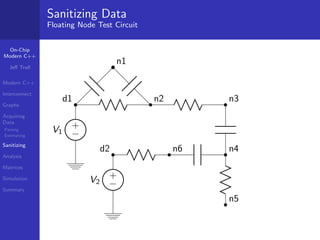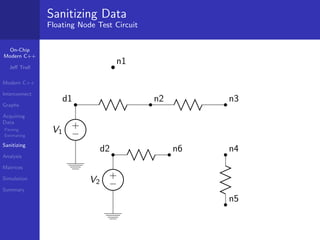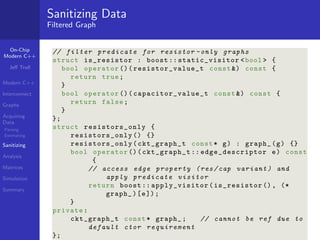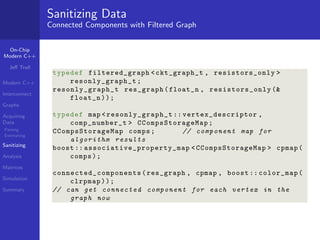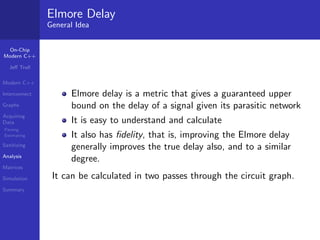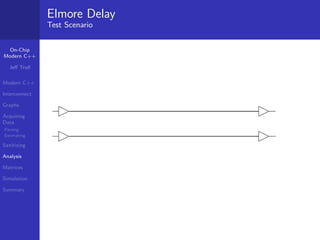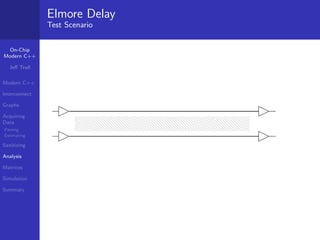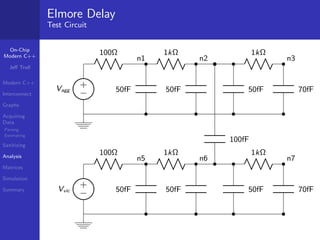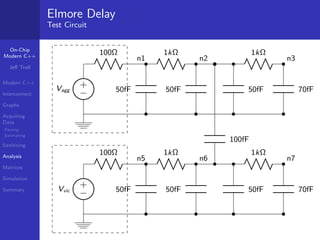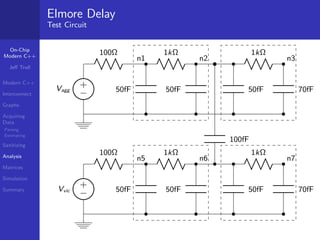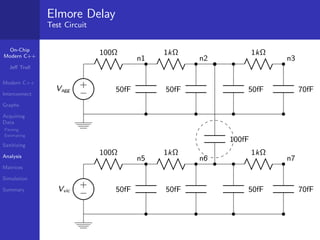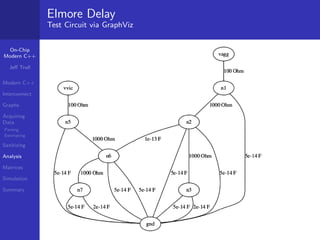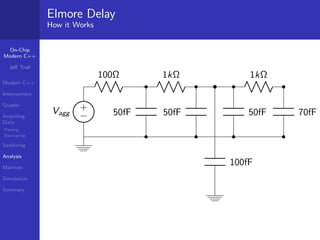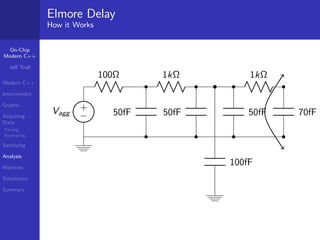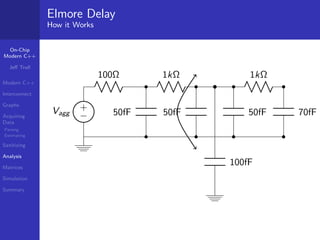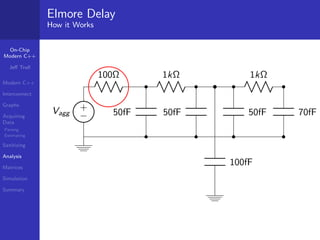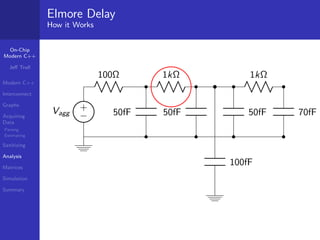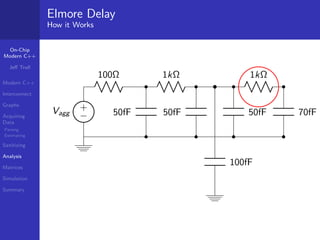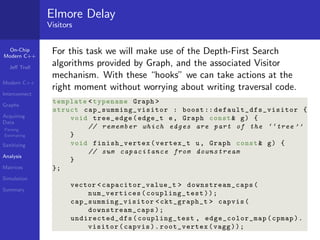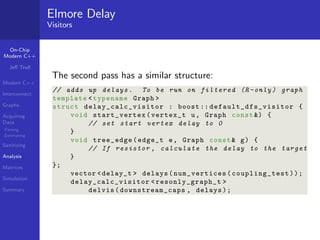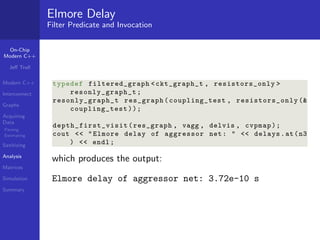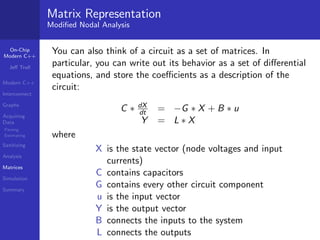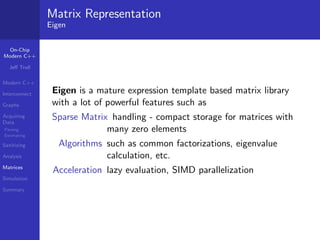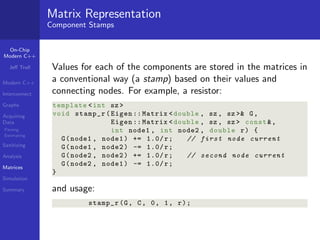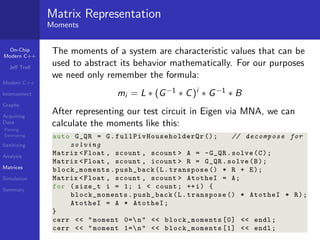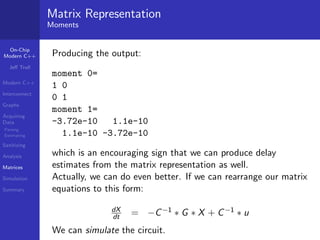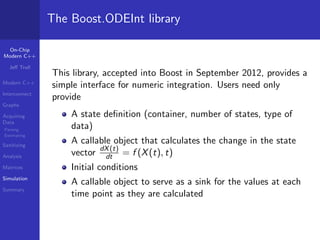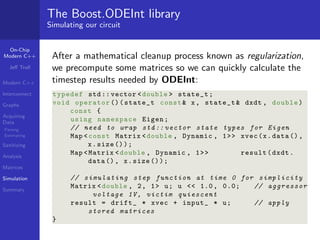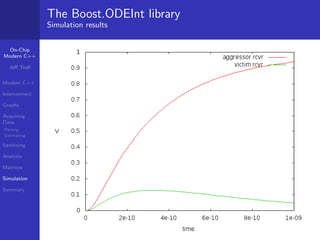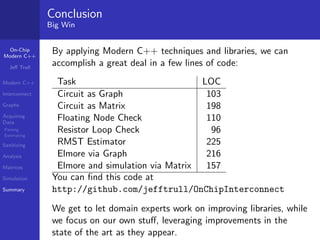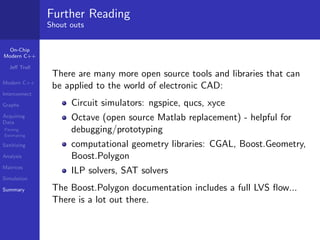1 of 62

### Analyzing On-Chip Interconnect with Modern C++

• 1. Analyzing On-Chip Interconnect with Modern C++ Je Trull October 12, 2014 c 2014 Jerey E. Trull Creative Commons 3.0 Attribution License
• 2. On-Chip Modern C++ Je Trull Modern C++ Interconnect Graphs Acquiring Data Parsing Estimating Sanitizing Analysis Matrices Simulation Summary Modern C++ In uences Functional Programming Generic Programming Design Patterns The Standard Library
• 3. On-Chip Modern C++ Je Trull Modern C++ Interconnect Graphs Acquiring Data Parsing Estimating Sanitizing Analysis Matrices Simulation Summary Modern C++ Is Concepts Iterators and Ranges Separation of Data and Algorithm Functions as First Class Objects More Declarative than Imperative Composition over Inheritance and reusable components that leverage those techniques . . .
• 4. On-Chip Modern C++ Je Trull Modern C++ Interconnect Graphs Acquiring Data Parsing Estimating Sanitizing Analysis Matrices Simulation Summary Modern C++ Probably Is Not C with Classes void * macros Idiosyncratic reimplementations of standard library features
• 5. On-Chip Modern C++ Je Trull Modern C++ Interconnect Graphs Acquiring Data Parsing Estimating Sanitizing Analysis Matrices Simulation Summary Connecting Logic Together You Need Wires Communication takes time and power proportional to the length of the wire.
• 6. On-Chip Modern C++ Je Trull Modern C++ Interconnect Graphs Acquiring Data Parsing Estimating Sanitizing Analysis Matrices Simulation Summary Connecting Logic Together You Need Wires Communication takes time and power proportional to the length of the wire. In addition, nearby wires may in uence the delay of the wire, or even produce an erroneous value.
• 7. On-Chip Modern C++ Je Trull Modern C++ Interconnect Graphs Acquiring Data Parsing Estimating Sanitizing Analysis Matrices Simulation Summary Circuit Analysis For Coders You can think of a circuit as a kind of graph. Edges are called branches and consist of a single component with a current that depends on its type, value, and the voltages of the nodes it connects Vertices usually called nodes, are simply connection points where currents ow between components R Node 1 Node 2 IR C IC Here IR = (VNode1 VNode2)=R, IC = C d VNode1VNode2 dt .
• 8. On-Chip Modern C++ Je Trull Modern C++ Interconnect Graphs Acquiring Data Parsing Estimating Sanitizing Analysis Matrices Simulation Summary Graph Storage The Boost.Graph library gives us several options for storing graphs: adjacency list The most popular; each vertex indexes a list of its adjacent vertices compressed sparse row Immutable but very compact adjacency matrix Represents graphs as matrices. Good for dense (many edges per vertex) graphs. Implicit - anything that behaves like a graph
• 9. On-Chip Modern C++ Je Trull Modern C++ Interconnect Graphs Acquiring Data Parsing Estimating Sanitizing Analysis Matrices Simulation Summary Graph Storage adjacency matrix one row, column per vertex 2z }| { 0 0 1 0 0 0 0 0 0 0 1 1 1 0 0 0 0 0 0 0 0 0 0 0 0 1 0 0 0 0 0 1 0 0 0 0 6666664 3 7777775 If the entry at M[i ; j ] is set, there is an edge from vertex i ! j . This gives constant time edge insertion and deletion at the cost of O(n2) memory usage. Foreshadowing There are subtle connections between graphs and matrices.
• 10. On-Chip Modern C++ Je Trull Modern C++ Interconnect Graphs Acquiring Data Parsing Estimating Sanitizing Analysis Matrices Simulation Summary Graph Storage Implicit graphs This can be anything that behaves like a graph, or more formally, models a Graph Concept, a set of expressions that must be valid when the class is used. Dierent graph algorithms place dierent requirements on the graphs they operate on. Typically when you create an implicit graph you do the minimum required for the algorithm you wish to use. Some things you might want to make an implicit Graph from: A social network's graph API A graph database Some other graph library's data A graph that is procedurally generated
• 11. On-Chip Modern C++ Je Trull Modern C++ Interconnect Graphs Acquiring Data Parsing Estimating Sanitizing Analysis Matrices Simulation Summary Graph Storage For Circuits I will use quantities from the Boost Units library for storing component values: Units Example using namespace boost :: units :: si; quantity resistance kohm (1.0 * kilo * ohms ); quantity capacitance ff (1.0 * femto * farads ); quantity time t = (2 * kohm ) * (6 * ff); std :: cout time constant =  t std :: endl ; time constant = 1.2e-11 s
• 12. On-Chip Modern C++ Je Trull Modern C++ Interconnect Graphs Acquiring Data Parsing Estimating Sanitizing Analysis Matrices Simulation Summary Graph Storage Vertex and Edge Properties We can assign arbitrary properties to vertices and edges, and access them through the Property Map mechanism. I'll store strings (node names) with the vertices. Edges, by contrast, can be one of two component types, which suggests: Boost.Variant typedef quantity resistance , double resistor_value_t ; typedef quantity capacitance , double capacitor_value_t ; typedef boost :: variant resistor_value_t , capacitor_value_t edge_property_t ;
• 13. On-Chip Modern C++ Je Trull Modern C++ Interconnect Graphs Acquiring Data Parsing Estimating Sanitizing Analysis Matrices Simulation Summary Graph Storage Boost.Variant A variant can store one of an arbitrary set of types in a safe, compile-time-checked manner: edge_property_t value = resistor_value_t (20.0* ohms ); // stores a resistor value = capacitor_value_t (17.0* ff); // now a capacitor resistor_value_t rvalue = get resistor_value_t ( value ); // throws - wrong type The recommended approach to accessing values is the visitor : struct cap_summer : boost :: static_visitor capacitor_value_t { capacitor_value_t operator ()( resistor_value_t const ) const { return capacitor_value_t (); // zero fF } capacitor_value_t operator ()( capacitor_value_t c) const { return c; } };
• 14. On-Chip Modern C++ Je Trull Modern C++ Interconnect Graphs Acquiring Data Parsing Estimating Sanitizing Analysis Matrices Simulation Summary Graph Storage De
• 15. ning and Using the Graph using namespace boost ; typedef adjacency_list vecS , vecS , undirectedS , vertex_property_t , edge_property_t ckt_graph_t ; ckt_graph_t circuit ; auto gnd = add_vertex ( gnd '', circuit ); auto in = add_vertex (in '', circuit ); auto out = add_vertex ( out '', circuit ); add_edge (in , out , 0.1* kohm , circuit ); add_edge (out , gnd , 20.0* ff , circuit ); We can view the results by using the build-in Graphviz writer: write_graphviz (dbg , circuit , make_label_writer ( get ( vertex_bundle , circuit )), make_label_writer ( get ( edge_bundle , circuit )));
• 16. On-Chip Modern C++ Je Trull Modern C++ Interconnect Graphs Acquiring Data Parsing Estimating Sanitizing Analysis Matrices Simulation Summary Acquiring Data SPEF The Standard Parasitic Exchange Format is a common choice for storing or transferring parasitic data between tools. It's relatively compact and fairly easy to parse (by design). We'll focus on two parts of the standard: The Name Map Allows us to abbreviate some potentially very long names: *NAME MAP *1 SOME/LONG/INSTANCE/PATH/n12888 *2 SOME/LONG/INSTANCE/PATH/n12889 ... Later expressions in the same
• 17. le may use *1, *2 in place of the full names
• 18. On-Chip Modern C++ Je Trull Modern C++ Interconnect Graphs Acquiring Data Parsing Estimating Sanitizing Analysis Matrices Simulation Summary Acquiring Data SPEF Parasitics for each net are split into resistors and capacitors and listed in a simple format: Component List *RES 1 *2172:Z *2173:1 0.230 2 *2173:1 *2173:2 18.1 ... *END We will demonstrate some simple parsing techniques for these two sections, using Boost.Spirit.
• 19. On-Chip Modern C++ Je Trull Modern C++ Interconnect Graphs Acquiring Data Parsing Estimating Sanitizing Analysis Matrices Simulation Summary Acquiring Data Parsing with Spirit The Spirit parser library overloads operators to turn expressions into parsers. If your needs are simple you can use those expressions directly: std :: string in(10 20 30 -11); vector int result ; auto beg = in. begin (); auto end = in. end (); qi :: phrase_parse (beg , end , // input range *int_ , // grammar ascii :: space , // skipper result ); // synthesized attribute copy ( result . begin () , result . end () , ostream_iterator int ( cout , )); cout endl ; outputs: 10 20 30 -11
• 20. On-Chip Modern C++ Je Trull Modern C++ Interconnect Graphs Acquiring Data Parsing Estimating Sanitizing Analysis Matrices Simulation Summary Acquiring Data Spirit : Symbol Table Spirit has a special component called symbols that can be loaded with data and then used as a parser. It is a sort of map, returning the data supplied as a value when the key is encountered in the input. symbols char , std :: string name_map_symtab ; typedef rule spirit :: istream_iterator , std :: string () name_rule ; name_rule nm_num = '*' + digit ; name_rule nm_name = + char_ (a-zA -Z0 -9/ _); namespace phx = boost :: phoenix ; phrase_parse (beg , end , lit (* NAME_MAP ) // grammar *( nm_num nm_name ) [ phx :: bind ( name_map_symtab .add , _1 , _2) // semantic action ], space );
• 21. On-Chip Modern C++ Je Trull Modern C++ Interconnect Graphs Acquiring Data Parsing Estimating Sanitizing Analysis Matrices Simulation Summary Acquiring Data Spirit : Using the Symbol Table Having loaded the name map, we can use it as a parser itself: name_rule node_with_symtab = '*' name_map_symtab char_ (':') + alnum ; phrase_parse (rbeg , rend , lit (*RES) *( omit [ uint_ ] node_with_symtab node_with_symtab double_ )[ phx :: bind ( ckt_builder :: add_component , phx :: ref ( builder ), _1 , _2 , _3 * phx :: cref ( r_unit ) )] lit (* END ), space );
• 22. On-Chip Modern C++ Je Trull Modern C++ Interconnect Graphs Acquiring Data Parsing Estimating Sanitizing Analysis Matrices Simulation Summary Acquiring Data Estimating Parasitics Sometimes it is valuable to create an approximate circuit for a signal that does not yet have parasitic information. Starting with the locations of the endpoints, a tree can be constructed using various heuristics. One of the simplest to implement is: The Rectilinear Minimum Spanning Tree Uses a Manhattan distance metric to select a set of connected edges with minimum cost Requires an initial graph containing all the candidate edges
• 23. On-Chip Modern C++ Je Trull Modern C++ Interconnect Graphs Acquiring Data Parsing Estimating Sanitizing Analysis Matrices Simulation Summary Acquiring Data RMST: Strategy We will: Create an implicit graph exposing all possible pairs of edges Create an implicit property map with the Manhattan metric for edges Apply the Boost.Graph supplied Prim MST algorithm
• 24. On-Chip Modern C++ Je Trull Modern C++ Interconnect Graphs Acquiring Data Parsing Estimating Sanitizing Analysis Matrices Simulation Summary Acquiring Data RMST: Implicit Graph We need a class to wrap a list of points and model the Graph concept. In practice, this means a collection of typedefs and free functions, plus our own (small) logic, e.g.: struct pin_distance_graph { typedef std :: pair int , int point_t ; template typename PtIter pin_distance_graph ( PtIter beg , PtIter end ) : points_ ( beg , end) {} // ( Some of the ) Requirements typedef size_t vertex_descriptor ; typedef std :: pair size_t , size_t edge_descriptor ; typedef boost :: undirected_tag directed_category ; friend vertices_size_type num_vertices ( pin_distance_graph const g) { return g. points_ . size (); } private : std :: vector point_t points_ ; };
• 25. On-Chip Modern C++ Je Trull Modern C++ Interconnect Graphs Acquiring Data Parsing Estimating Sanitizing Analysis Matrices Simulation Summary Acquiring Data RMST: Implicit Edge Weight Map We don't need to store a map containing distances for all possible vertex pairs, because we can calculate them on demand instead: typedef pin_distance_graph :: edge_descriptor edge_t ; auto weightpmap = make_function_property_map edge_t ( [ pdg ]( edge_t e) - int { auto coord1 = pdg [ source (e, pdg )]; auto coord2 = pdg [ target (e, pdg )]; return abs ( coord1 . first - coord2 . first ) + abs ( coord1 . second - coord2 . second ); });
• 26. On-Chip Modern C++ Je Trull Modern C++ Interconnect Graphs Acquiring Data Parsing Estimating Sanitizing Analysis Matrices Simulation Summary Acquiring Data RMST: Call Prim, make output // Predecessor map vector pin_distance_graph :: vertex_descriptor predvec ( num_vertices (pdg)); // underlying storage auto predpmap = make_iterator_property_map ( predvec . begin () , vindex_map ); // Calculate MST prim_minimum_spanning_tree (pdg , predpmap , weight_map ( weightpmap ). vertex_index_map ( vindex_map )); // Output auto vitpair = vertices ( pdg ); for ( auto v : make_iterator_range ( vitpair .first , vitpair . second )) { if ( predvec [v] == v) { // root node } else { // MST edge between v and its predecessor } }
• 27. On-Chip Modern C++ Je Trull Modern C++ Interconnect Graphs Acquiring Data Parsing Estimating Sanitizing Analysis Matrices Simulation Summary RMST Example
• 28. On-Chip Modern C++ Je Trull Modern C++ Interconnect Graphs Acquiring Data Parsing Estimating Sanitizing Analysis Matrices Simulation Summary RMST Complete Graph
• 29. On-Chip Modern C++ Je Trull Modern C++ Interconnect Graphs Acquiring Data Parsing Estimating Sanitizing Analysis Matrices Simulation Summary RMST Result
• 30. On-Chip Modern C++ Je Trull Modern C++ Interconnect Graphs Acquiring Data Parsing Estimating Sanitizing Analysis Matrices Simulation Summary RMST Further Optimizations How to perform this kind of estimate well is a popular research topic. RMST is easy to calculate but produces trees up to 50% more costly than optimal. Other approaches include: The Steiner tree, where additional, optional vertices are added to the graph. This is an NP-hard problem but there are many published algorithms. Starting with a Delaunay triangulation instead of a complete graph, to improve runtime (see Boost.Polygon) Using a maze router to improve estimates in the presence of blockages
• 31. On-Chip Modern C++ Je Trull Modern C++ Interconnect Graphs Acquiring Data Parsing Estimating Sanitizing Analysis Matrices Simulation Summary Sanitizing Input Generated parasitics from complex designs can have a number of issues that produce problems for tools, including: Resistor loops Floating (undriven) nodes Components of very small value It's useful to be able to recognize and/or repair these cases, as problems often manifest themselves as bad math results, with poor diagnostics.
• 32. On-Chip Modern C++ Je Trull Modern C++ Interconnect Graphs Acquiring Data Parsing Estimating Sanitizing Analysis Matrices Simulation Summary Sanitizing Data Floating Node Detection If a circuit node has no path strictly through resistors to either a driving cell, or to the ground node, there is nothing really controlling its voltage, so we say it is oating. Electrically this is a bad thing, but it can happen at intermediate stages in a design when the chip wiring is incomplete. Strategy We will Use a
• 33. ltered Graph to produce a view of the circuit with only resistor edges Apply the connected components algorithm to group circuit nodes with their driving cell
• 34. On-Chip Modern C++ Je Trull Modern C++ Interconnect Graphs Acquiring Data Parsing Estimating Sanitizing Analysis Matrices Simulation Summary Sanitizing Data Floating Node Test Circuit + V1 d1 n2 n3 + V2 d2 n6 n4 n5 n1
• 35. On-Chip Modern C++ Je Trull Modern C++ Interconnect Graphs Acquiring Data Parsing Estimating Sanitizing Analysis Matrices Simulation Summary Sanitizing Data Floating Node Test Circuit + V1 d1 n2 n3 + V2 d2 n6 n4 n5 n1
• 36. On-Chip Modern C++ Je Trull Modern C++ Interconnect Graphs Acquiring Data Parsing Estimating Sanitizing Analysis Matrices Simulation Summary Sanitizing Data Filtered Graph // filter predicate for resistor - only graphs struct is_resistor : boost :: static_visitor bool { bool operator ()( resistor_value_t const ) const { return true ; } bool operator ()( capacitor_value_t const ) const { return false ; } }; struct resistors_only { resistors_only () {} resistors_only ( ckt_graph_t const * g) : graph_ (g) {} bool operator ()( ckt_graph_t :: edge_descriptor e) const { // access edge property ( res / cap variant ) and apply predicate visitor return boost :: apply_visitor ( is_resistor () , (* graph_ )[e]); } private : ckt_graph_t const * graph_ ; // cannot be ref due to default ctor requirement };
• 37. On-Chip Modern C++ Je Trull Modern C++ Interconnect Graphs Acquiring Data Parsing Estimating Sanitizing Analysis Matrices Simulation Summary Sanitizing Data Connected Components with Filtered Graph typedef filtered_graph ckt_graph_t , resistors_only resonly_graph_t ; resonly_graph_t res_graph ( float_n , resistors_only ( float_n )); typedef map resonly_graph_t :: vertex_descriptor , comp_number_t CCompsStorageMap ; CCompsStorageMap comps ; // component map for algorithm results boost :: associative_property_map CCompsStorageMap cpmap ( comps ); connected_components ( res_graph , cpmap , boost :: color_map ( clrpmap )); // can get connected component for each vertex in the graph now
• 38. On-Chip Modern C++ Je Trull Modern C++ Interconnect Graphs Acquiring Data Parsing Estimating Sanitizing Analysis Matrices Simulation Summary Elmore Delay General Idea Elmore delay is a metric that gives a guaranteed upper bound on the delay of a signal given its parasitic network It is easy to understand and calculate It also has
• 39. delity, that is, improving the Elmore delay generally improves the true delay also, and to a similar degree. It can be calculated in two passes through the circuit graph.
• 40. On-Chip Modern C++ Je Trull Modern C++ Interconnect Graphs Acquiring Data Parsing Estimating Sanitizing Analysis Matrices Simulation Summary Elmore Delay Test Scenario
• 41. On-Chip Modern C++ Je Trull Modern C++ Interconnect Graphs Acquiring Data Parsing Estimating Sanitizing Analysis Matrices Simulation Summary Elmore Delay Test Scenario
• 42. On-Chip Modern C++ Je Trull Modern C++ Interconnect Graphs Acquiring Data Parsing Estimating Sanitizing Analysis Matrices Simulation Summary Elmore Delay Test Circuit + Vagg 100 1k 1k n1 n2 n3 50fF 50fF 50fF 70fF + Vvic 100fF 100 1k 1k n5 n6 n7 50fF 50fF 50fF 70fF
• 43. On-Chip Modern C++ Je Trull Modern C++ Interconnect Graphs Acquiring Data Parsing Estimating Sanitizing Analysis Matrices Simulation Summary Elmore Delay Test Circuit + Vagg 100 1k 1k n1 n2 n3 50fF 50fF 50fF 70fF + Vvic 100fF 100 1k 1k n5 n6 n7 50fF 50fF 50fF 70fF
• 44. On-Chip Modern C++ Je Trull Modern C++ Interconnect Graphs Acquiring Data Parsing Estimating Sanitizing Analysis Matrices Simulation Summary Elmore Delay Test Circuit + Vagg 100 1k 1k n1 n2 n3 50fF 50fF 50fF 70fF + Vvic 100fF 100 1k 1k n5 n6 n7 50fF 50fF 50fF 70fF
• 45. On-Chip Modern C++ Je Trull Modern C++ Interconnect Graphs Acquiring Data Parsing Estimating Sanitizing Analysis Matrices Simulation Summary Elmore Delay Test Circuit + Vagg 100 1k 1k n1 n2 n3 50fF 50fF 50fF 70fF + Vvic 100fF 100 1k 1k n5 n6 n7 50fF 50fF 50fF 70fF
• 46. On-Chip Modern C++ Je Trull Modern C++ Interconnect Graphs Acquiring Data Parsing Estimating Sanitizing Analysis Matrices Simulation Summary Elmore Delay Test Circuit via GraphViz
• 47. On-Chip Modern C++ Je Trull Modern C++ Interconnect Graphs Acquiring Data Parsing Estimating Sanitizing Analysis Matrices Simulation Summary Elmore Delay How it Works + Vagg 100 1k 1k 50fF 50fF 50fF 70fF 100fF
• 48. On-Chip Modern C++ Je Trull Modern C++ Interconnect Graphs Acquiring Data Parsing Estimating Sanitizing Analysis Matrices Simulation Summary Elmore Delay How it Works + Vagg 100 1k 1k 50fF 50fF 50fF 70fF 100fF
• 49. On-Chip Modern C++ Je Trull Modern C++ Interconnect Graphs Acquiring Data Parsing Estimating Sanitizing Analysis Matrices Simulation Summary Elmore Delay How it Works + Vagg 100 1k 1k 50fF 50fF 50fF 70fF 100fF
• 50. On-Chip Modern C++ Je Trull Modern C++ Interconnect Graphs Acquiring Data Parsing Estimating Sanitizing Analysis Matrices Simulation Summary Elmore Delay How it Works + Vagg 100 1k 1k 50fF 50fF 50fF 70fF 100fF
• 51. On-Chip Modern C++ Je Trull Modern C++ Interconnect Graphs Acquiring Data Parsing Estimating Sanitizing Analysis Matrices Simulation Summary Elmore Delay How it Works + Vagg 100 1k 1k 50fF 50fF 50fF 70fF 100fF
• 52. On-Chip Modern C++ Je Trull Modern C++ Interconnect Graphs Acquiring Data Parsing Estimating Sanitizing Analysis Matrices Simulation Summary Elmore Delay How it Works + Vagg 100 1k 1k 50fF 50fF 50fF 70fF 100fF
• 53. On-Chip Modern C++ Je Trull Modern C++ Interconnect Graphs Acquiring Data Parsing Estimating Sanitizing Analysis Matrices Simulation Summary Elmore Delay How it Works + Vagg 100 1k 1k 50fF 50fF 50fF 70fF 100fF
• 54. On-Chip Modern C++ Je Trull Modern C++ Interconnect Graphs Acquiring Data Parsing Estimating Sanitizing Analysis Matrices Simulation Summary Elmore Delay Visitors For this task we will make use of the Depth-First Search algorithms provided by Graph, and the associated Visitor mechanism. With these hooks we can take actions at the right moment without worrying about writing traversal code. template typename Graph struct cap_summing_visitor : boost :: default_dfs_visitor { void tree_edge ( edge_t e, Graph const g) { // remember which edges are part of the  tree '' } void finish_vertex ( vertex_t u, Graph const g) { // sum capacitance from downstream } }; vector capacitor_value_t downstream_caps ( num_vertices ( coupling_test )); cap_summing_visitor ckt_graph_t capvis ( downstream_caps ); undirected_dfs ( coupling_test , edge_color_map ( cpmap ). visitor ( capvis ). root_vertex ( vagg ));
• 55. On-Chip Modern C++ Je Trull Modern C++ Interconnect Graphs Acquiring Data Parsing Estimating Sanitizing Analysis Matrices Simulation Summary Elmore Delay Visitors The second pass has a similar structure: // adds up delays . To be run on filtered (R- only ) graph template typename Graph struct delay_calc_visitor : boost :: default_dfs_visitor { void start_vertex ( vertex_t u, Graph const ) { // set start vertex delay to 0 } void tree_edge ( edge_t e, Graph const g) { // If resistor , calculate the delay to the target } }; vector delay_t delays ( num_vertices ( coupling_test )); delay_calc_visitor resonly_graph_t delvis ( downstream_caps , delays );
• 56. On-Chip Modern C++ Je Trull Modern C++ Interconnect Graphs Acquiring Data Parsing Estimating Sanitizing Analysis Matrices Simulation Summary Elmore Delay Filter Predicate and Invocation typedef filtered_graph ckt_graph_t , resistors_only resonly_graph_t ; resonly_graph_t res_graph ( coupling_test , resistors_only ( coupling_test )); depth_first_visit ( res_graph , vagg , delvis , cvpmap ); cout Elmore delay of aggressor net : delays .at(n3 ) endl ; which produces the output: Elmore delay of aggressor net: 3.72e-10 s
• 57. On-Chip Modern C++ Je Trull Modern C++ Interconnect Graphs Acquiring Data Parsing Estimating Sanitizing Analysis Matrices Simulation Summary Matrix Representation Modi
• 58. ed Nodal Analysis You can also think of a circuit as a set of matrices. In particular, you can write out its behavior as a set of dierential equations, and store the coecients as a description of the circuit: C dX dt = G X + B u Y = L X where X is the state vector (node voltages and input currents) C contains capacitors G contains every other circuit component u is the input vector Y is the output vector B connects the inputs to the system L connects the outputs
• 59. On-Chip Modern C++ Je Trull Modern C++ Interconnect Graphs Acquiring Data Parsing Estimating Sanitizing Analysis Matrices Simulation Summary Matrix Representation Eigen Eigen is a mature expression template based matrix library with a lot of powerful features such as Sparse Matrix handling - compact storage for matrices with many zero elements Algorithms such as common factorizations, eigenvalue calculation, etc. Acceleration lazy evaluation, SIMD parallelization
• 60. On-Chip Modern C++ Je Trull Modern C++ Interconnect Graphs Acquiring Data Parsing Estimating Sanitizing Analysis Matrices Simulation Summary Matrix Representation Component Stamps Values for each of the components are stored in the matrices in a conventional way (a stamp) based on their values and connecting nodes. For example, a resistor: template int sz void stamp_r ( Eigen :: Matrix double , sz , sz G, Eigen :: Matrix double , sz , sz const , int node1 , int node2 , double r) { G(node1 , node1 ) += 1.0/ r; // first node current G(node1 , node2 ) -= 1.0/ r; G(node2 , node2 ) += 1.0/ r; // second node current G(node2 , node1 ) -= 1.0/ r; } and usage: stamp_r (G, C, 0, 1, r);
• 61. On-Chip Modern C++ Je Trull Modern C++ Interconnect Graphs Acquiring Data Parsing Estimating Sanitizing Analysis Matrices Simulation Summary Matrix Representation Moments The moments of a system are characteristic values that can be used to abstract its behavior mathematically. For our purposes we need only remember the formula: mi = L (G1 C)i G1 B After representing our test circuit in Eigen via MNA, we can calculate the moments like this: auto G_QR = G. fullPivHouseholderQr (); // decompose for solving Matrix Float , scount , scount A = -G_QR . solve (C); Matrix Float , scount , icount R = G_QR . solve (B); block_moments . push_back (L. transpose () * R + E); Matrix Float , scount , scount AtotheI = A; for ( size_t i = 1; i count ; ++i) { block_moments . push_back (L. transpose () * AtotheI * R); AtotheI = A * AtotheI ; } cerr moment 0= n block_moments  endl ; cerr moment 1= n block_moments  endl ;
• 62. On-Chip Modern C++ Je Trull Modern C++ Interconnect Graphs Acquiring Data Parsing Estimating Sanitizing Analysis Matrices Simulation Summary Matrix Representation Moments Producing the output: moment 0= 1 0 0 1 moment 1= -3.72e-10 1.1e-10 1.1e-10 -3.72e-10 which is an encouraging sign that we can produce delay estimates from the matrix representation as well. Actually, we can do even better. If we can rearrange our matrix equations to this form: dX dt = C1 G X + C1 u We can simulate the circuit.
• 63. On-Chip Modern C++ Je Trull Modern C++ Interconnect Graphs Acquiring Data Parsing Estimating Sanitizing Analysis Matrices Simulation Summary The Boost.ODEInt library This library, accepted into Boost in September 2012, provides a simple interface for numeric integration. Users need only provide A state de
• 64. nition (container, number of states, type of data) A callable object that calculates the change in the state vector dX(t) dt = f (X(t); t) Initial conditions A callable object to serve as a sink for the values at each time point as they are calculated
• 65. On-Chip Modern C++ Je Trull Modern C++ Interconnect Graphs Acquiring Data Parsing Estimating Sanitizing Analysis Matrices Simulation Summary The Boost.ODEInt library Simulating our circuit After a mathematical cleanup process known as regularization, we precompute some matrices so we can quickly calculate the timestep results needed by ODEInt: typedef std :: vector double state_t ; void operator ()( state_t const x, state_t dxdt , double ) const { using namespace Eigen ; // need to wrap std :: vector state types for Eigen Map const Matrix double , Dynamic , 1 xvec (x. data () , x. size ()); Map Matrix double , Dynamic , 1 result ( dxdt . data () , x. size ()); // simulating step function at time 0 for simplicity Matrix double , 2, 1 u; u 1.0 , 0.0; // aggressor voltage 1V, victim quiescent result = drift_ * xvec + input_ * u; // apply stored matrices }
• 66. On-Chip Modern C++ Je Trull Modern C++ Interconnect Graphs Acquiring Data Parsing Estimating Sanitizing Analysis Matrices Simulation Summary The Boost.ODEInt library Simulation results
• 67. On-Chip Modern C++ Je Trull Modern C++ Interconnect Graphs Acquiring Data Parsing Estimating Sanitizing Analysis Matrices Simulation Summary Conclusion Big Win By applying Modern C++ techniques and libraries, we can accomplish a great deal in a few lines of code: Task LOC Circuit as Graph 103 Circuit as Matrix 198 Floating Node Check 110 Resistor Loop Check 96 RMST Estimator 225 Elmore via Graph 216 Elmore and simulation via Matrix 157 You can
• 68. nd this code at http://github.com/jefftrull/OnChipInterconnect We get to let domain experts work on improving libraries, while we focus on our own stu, leveraging improvements in the state of the art as they appear.
• 69. On-Chip Modern C++ Je Trull Modern C++ Interconnect Graphs Acquiring Data Parsing Estimating Sanitizing Analysis Matrices Simulation Summary Further Reading Shout outs There are many more open source tools and libraries that can be applied to the world of electronic CAD: Circuit simulators: ngspice, qucs, xyce Octave (open source Matlab replacement) - helpful for debugging/prototyping computational geometry libraries: CGAL, Boost.Geometry, Boost.Polygon ILP solvers, SAT solvers The Boost.Polygon documentation includes a full LVS ow... There is a lot out there.